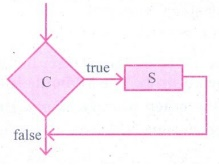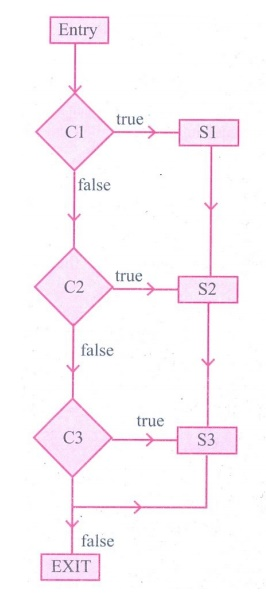Home | | Computer Science 11th std | Answer the following questions

## Chapter: 11th Computer Science : Chapter 7 : Composition and Decomposition

Short Answers, Explain in Brief, Explain in detail, Important Questions - Computer Science : Algorithmic Problem Solving : Composition and Decomposition

Computer Science: Algorithmic Problem Solving

Composition and Decomposition

Evaluation

Part II

1. Distinguish between a condition and a statement.Condition

(i) Condition is the checking process of either True/False.

(ii) A condition is contained in a diamond shaped box with two outgoing arrows, labeled true and false.

(iii) Ex : a > b

Statement

(i) Processing the condition.

(ii) A statement is contained in a rectangular box with a single outgoing arrow, which points to the box to be executed next.

(iii) Ex : Print a (a - is a biggest value).

2. Draw a flowchart for conditional statement.3. Both conditional statement and iterative statement have a condition and a statement. How do they differ?

Answer: Conditional statement is executed only if the condition is true. Otherwise, nothing is done.

Iterative statement repeatedly evaluates a condition and executes a statement as long as the condition is true.

4. What is the difference between an algorithm and a program?

Answer: An algorithm is a self-contained step-by-step set of operations to be performed to solve a specific problems. A computer program is a sequence of instructions that complete the rules of a specific programming language, written to perform a specified task with a computer.

5. Why is function an abstraction?

Answer: (i) The parts of an algorithm are known as functions. A function is like a sub algorithm.

(ii) It takes an input and produces an output, satisfying a desired input output relation.

6. How do we refine a statement?

Answer: In refinement, starting at a high level, each statement is repeatedly expanded into more detailed statements in the subsequent levels.

Part III

1. For the given two flowcharts write the pseudo code.1. Enter A, B

2. Initialize Q= 0, r = A

3. If r ≥ B, then do Q = Q+ 1; r = r - B else r, q

4. Exit.

2. If C is false in line 2, trace the control flow in this algorithm.

1 S1

2 -- C is false

3 if C

4 S2

5 else

6 S3

7 S4

3. What is case analysis?

Answer: Case analysis statement generalizes it to multiple cases. Case analysis splits the problem into an exhaustive set of disjoint cases.

4. Draw a flowchart for -3case analysis using alternative statements.5. Define a function to double a number in two different ways: (1) n + n, (2) 2 x n

(1) double (n)

-- inputs : n is a real number or an integer, n > 0

-- outputs : y is a real number or an integer such that y = n + n

(2) double (n)

-- inputs : n is a real number or an integer, n > 0

-- outputs : y is a real number or an integer such that y = 2 × n.

Part IV

1. Exchange the contents: Given two glasses marked A and B. Glass A is full of apple drink and glass B is full of grape drink. Write the specification forexchanging the contents of glasses A and B, and write a sequence of assignments to satisfy the specification.

Exchange (A, B)

-- inputs : A, B are real number or an integers,

A≠ 0, B ≠ 0

-- outputs : t is an integer such that

t :=A; A:=B; B:=t.

2. Circulate the contents: Write the specification and construct an algorithm to circulate the contents of the variables A, B and C as shown below: The arrows indicate that B gets the value of A, C gets the value of B and A gets the value of C.Circulate (A, B, C)

-- inputs : A, B, C are real numbers or an integers,

A≠0, B≠0, C≠0

-- outputs : tl:= B; t2:=C such that

B:=A; C:=tl; A:= t2;

3. Decanting problem. You are given three bottles of capacities 5 ,8, and 3 litres. The 8L bottle is filled with oil, while the other two are empty. Divide the oil in 8L bottle into two equal quantities. Represent the state of the process by appropriate variables. What are the initial and final states of the process? Model the decanting of oil from one bottle to another by assignment. Write a sequence of assignments to achieve the final state.

1. A := 8, B:=0, C:=0

2. E, F, T:=A, B, C

3. F: = E−3

4. T:=F−3

5. E: = E + T

6. T: = F

F: = F−2

7. F: = E−1

8. F : = F −1

T: = T+ 1

9. E : = E + T

T : = T − 3

=========

E F T

1. 8, 0, 0

2. 3, 5, 0

3. 3, 2, 3

4.

3+3

E F T

6 2 0

1, 5, 2

1, 4, 3

4, 4, 0

4. Trace the step-by-step execution of the algorithm for factorial(4).

factorial(n)

--inputs : n is an integer , n ≥ 0

--outputs : f = n!

f, i := 1 ,1

while i ≤ n

f, i := f × i, i+1

factorial (4)

i= l,f=1;

= f= l × 1

= f = 1 × 2

= f = 2 × 3

= f = 6 × 4

= f = 24

Tags : Composition and Decomposition | Computer Science , 11th Computer Science : Chapter 7 : Composition and Decomposition
Study Material, Lecturing Notes, Assignment, Reference, Wiki description explanation, brief detail
11th Computer Science : Chapter 7 : Composition and Decomposition : Answer the following questions | Composition and Decomposition | Computer Science